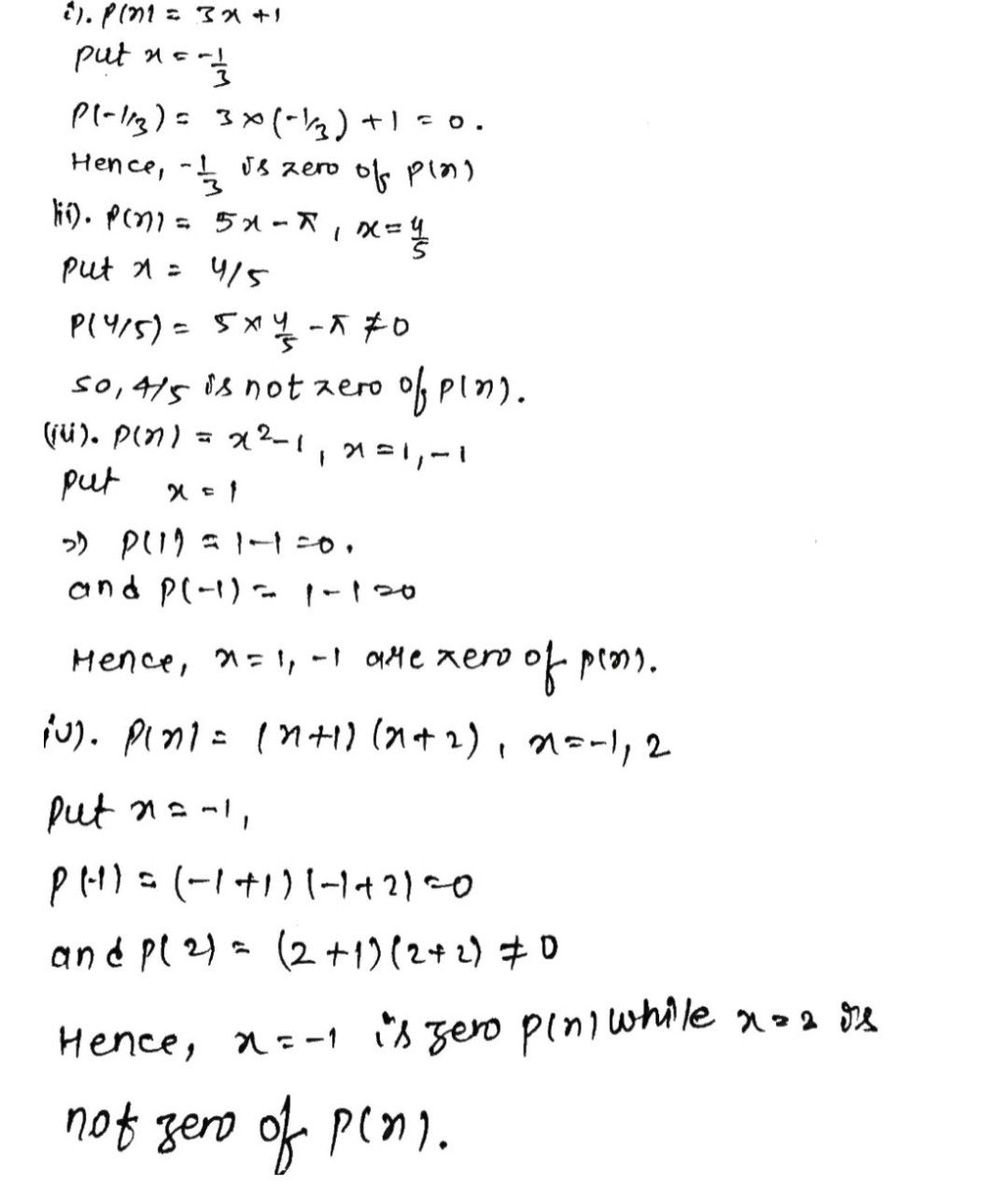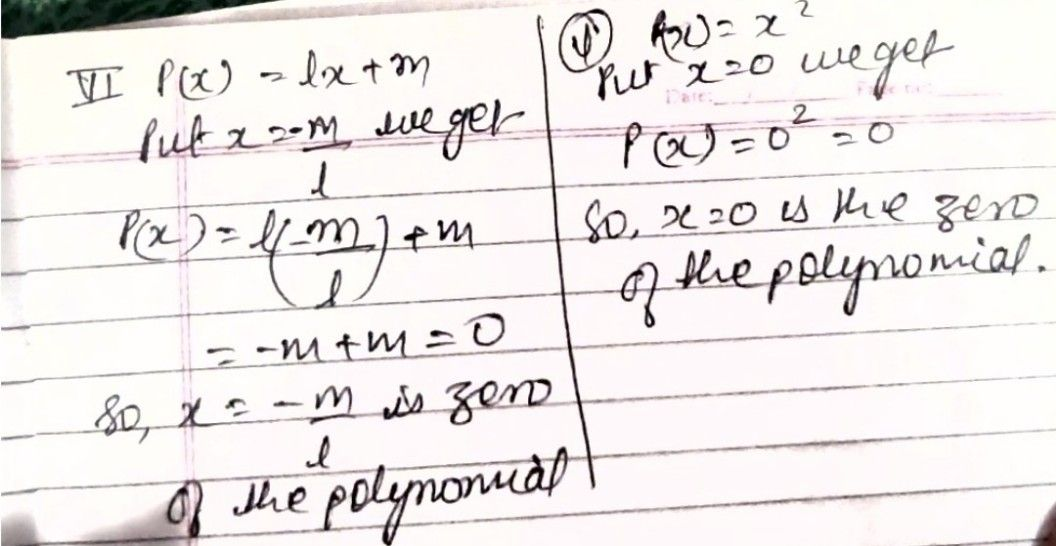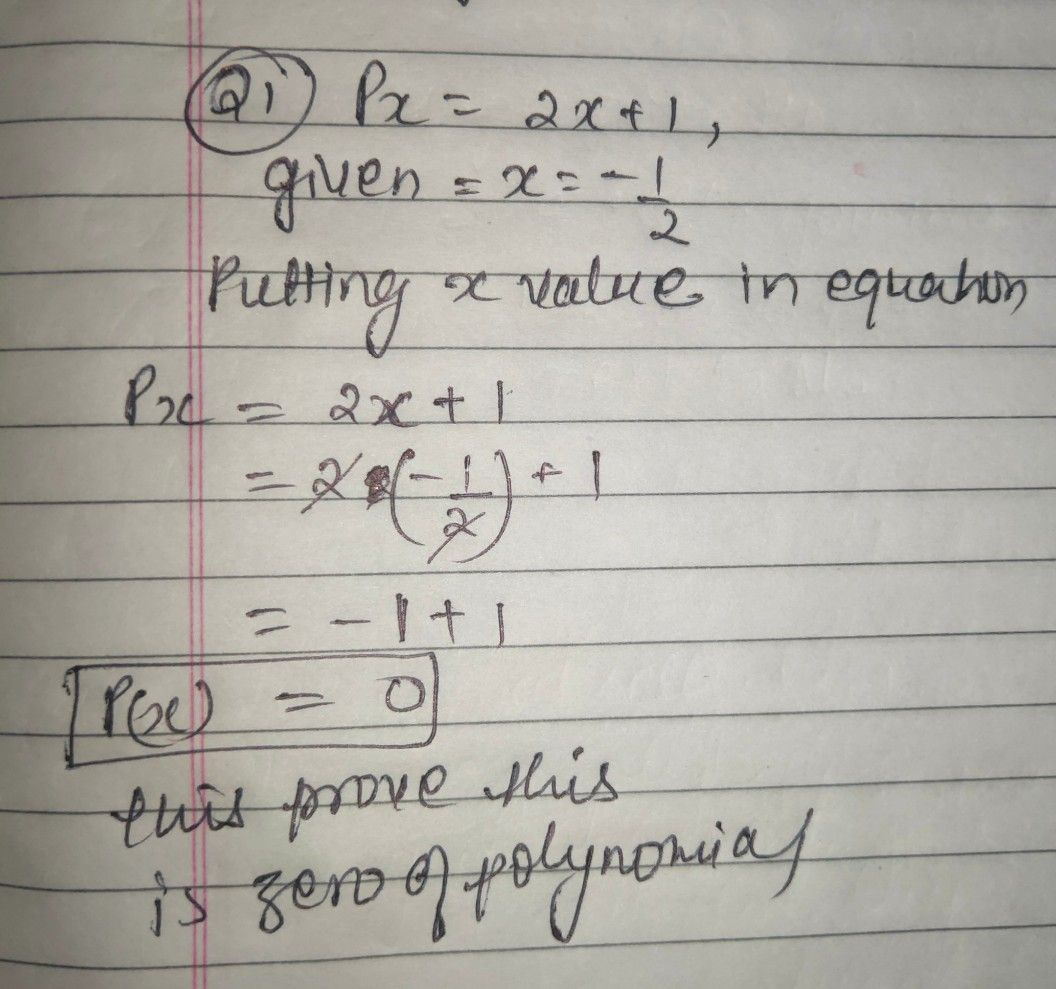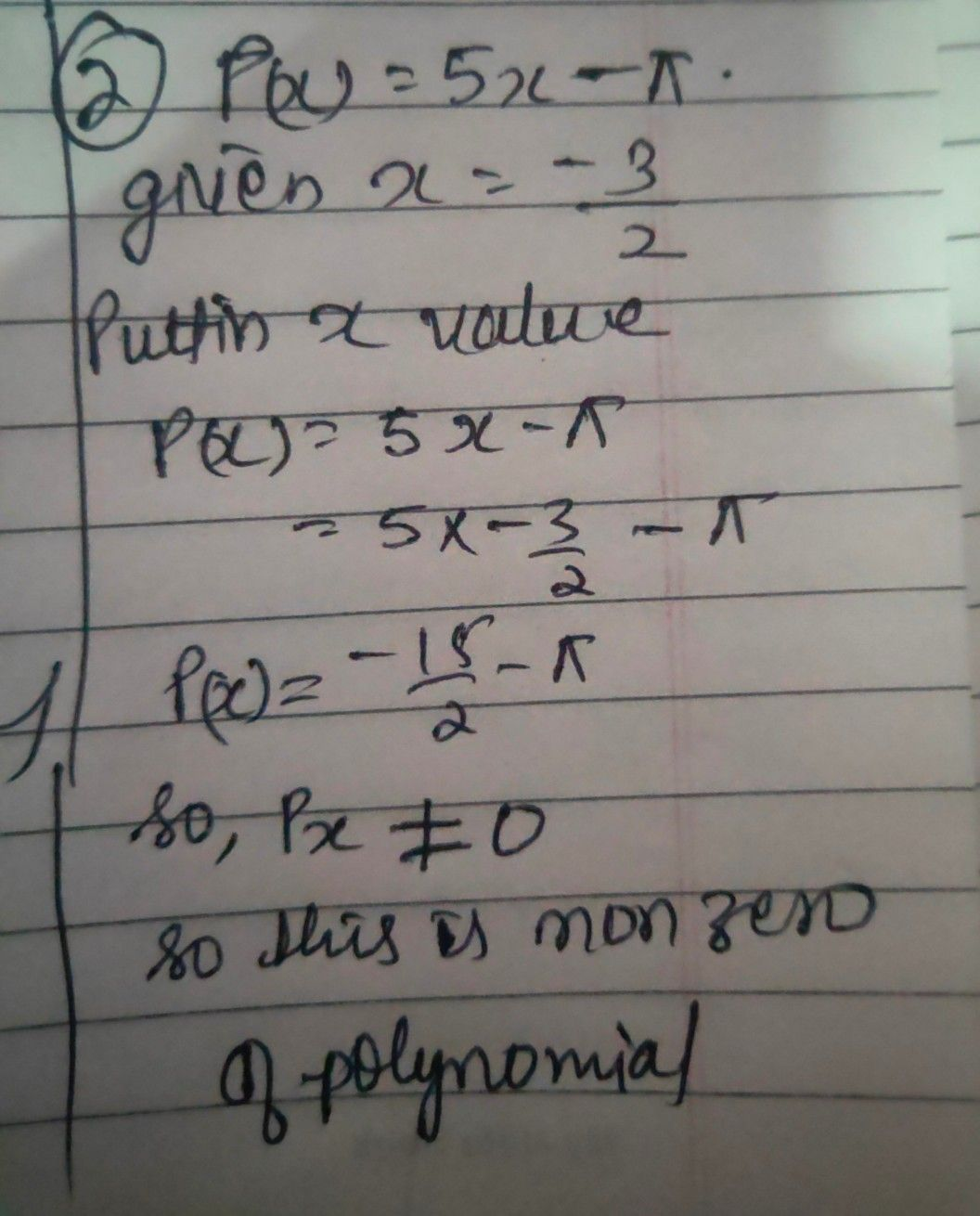Symbol
Problem$3$ Verify whether the values of x given in each case are the zeroes of the polynomial or not?. $\left(1\right)$ $p\left(x\right)=2x+1:x=-\dfrac {1} {2}$ $\left(i\right)$ $p\left(x\right)=5x-\pi :x=\dfrac {-3} {2}$ $\left(1i1\right)$ $p\left(x\right)=x^{2}-1:x=±1$ $\left(iy\right)$ $p\left(x\right)=\left(x-1\right)\left(x+2\right):x=-1,-2$ $\left(v\right)$ $P\left(y\right)=y^{2}:y=0$ $\left(n\right)$ $p\left(x\right)=ax+b:x=-\dfrac {b} {a}$ $1$ $2$ $1$ 1 $-1$
7th-9th grade
Algebra
Search count: 105
SolutionQanda teacher - sameeraStudent
thank u once again maamQanda teacher - sameera
??
plz evaluate and also give extra coins as thankyou gift ??
now u get how to ask in preferred
soo keep asking I'm always here to help u
?Student
ma'am have u recived 1000 coins gift from meQanda teacher - sameera
no
only 750 gift coins u have given me
?Student
I think I did a mistake
while sending giftQanda teacher - sameera
ohh
ok send this time
plz evaluate and also give extra coins as thankyou gift ??Student
on 18th I have math test please help meQanda teacher - sameera
Yes offcourseStudent
the potion is polynomial and factorisationQanda teacher - sameera
ok
i can ask me any questions
in preferredStudent
you have given me only solutions
explain me
how to solve itQanda teacher - sameera
ok
in your QUESTION to find zero of polynomials
that means p(x)=0
in ur question no. 1Student
you can just record a video and send itQanda teacher - sameera
qanda had no feature like thi
video recording cannot occurStudent
ohhQanda teacher - sameera
yesStudent
so we will continue like this only maamQanda teacher - sameera
yesStudent
ma'am continue explaining question no1Qanda teacher - sameeraStudent
thank u maamQanda teacher - sameeranow u get it
by this method u can do all questions like thisStudent
ma'am do u live in hyderabadQanda teacher - sameera
plz no personal questionStudent
do u take tuitionsQanda teacher - sameera
noStudent
Can u send me your phone number so I can call u and clear my doubtsQanda teacher - sameera
no
sharing any personal information is not allowedStudent
ma'am I am weak at maths I need your helpQanda teacher - sameera
so u can ask in qanda any time
i will give u ans
i cant help it more than thatStudent
ok then and thank u once again maamQanda teacher - sameera
ok
plz evaluate and also give extra coins as thankyou gift ??Student
and sorry for asking personal questionsQanda teacher - sameera
its ok?Student
your so nice maamQanda teacher - sameera
?
plz evaluate and also give extra coins as thankyou gift ??Student
bye ma'am you really helped me a lot good night sweet dreamsQanda teacher - sameera
okStudent
???Qanda teacher - sameera
plz evaluate and also give extra coins as thankyou gift ??